# Chapter 7 Expectation 7 1 Mathematical expectation 7

• Slides: 13Chapter 7 Expectation 7. 1 Mathematical expectation7. 1 Mathematical Expectation Mathematical expectation =expected long run average Simulation 1: toss a fair coin H 1, T 0. n=10 times: 1 0 1 Average=0. 7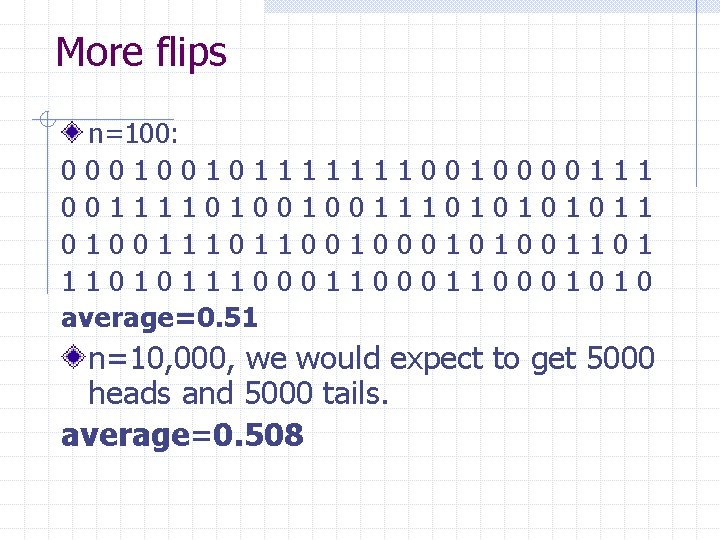More flips n=100: 000100101 001111010 01001110101110 average=0. 51 1 0 1 1 0 0 1 1 0 0 0 0 1 1 0 1 0 0 0 0 1 1 0 1 1 0 n=10, 000, we would expect to get 5000 heads and 5000 tails. average=0. 508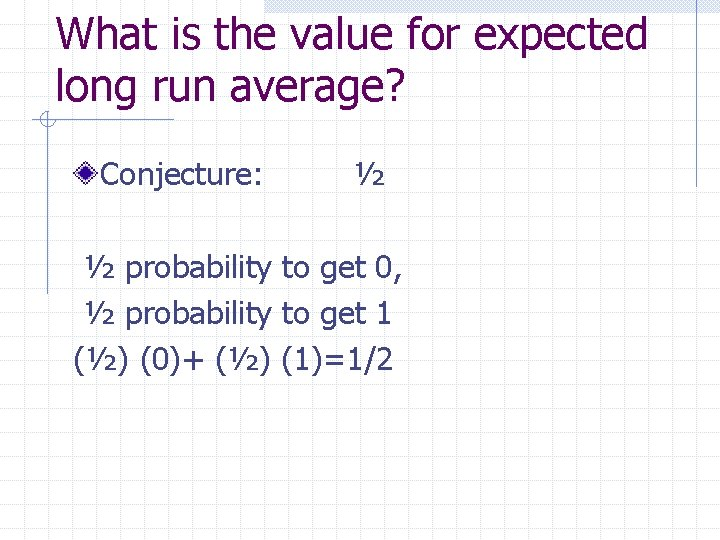What is the value for expected long run average? Conjecture: ½ ½ probability to get 0, ½ probability to get 1 (½) (0)+ (½) (1)=1/2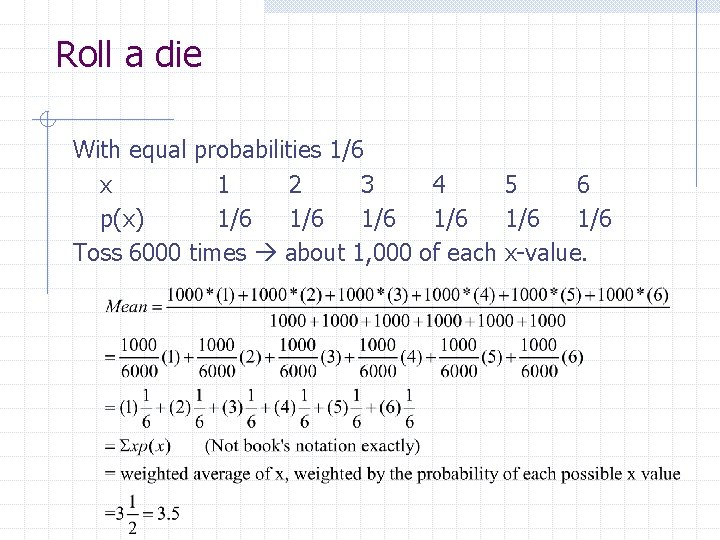Roll a die With equal probabilities 1/6 x 1 2 3 4 5 6 p(x) 1/6 1/6 1/6 Toss 6000 times about 1, 000 of each x-value.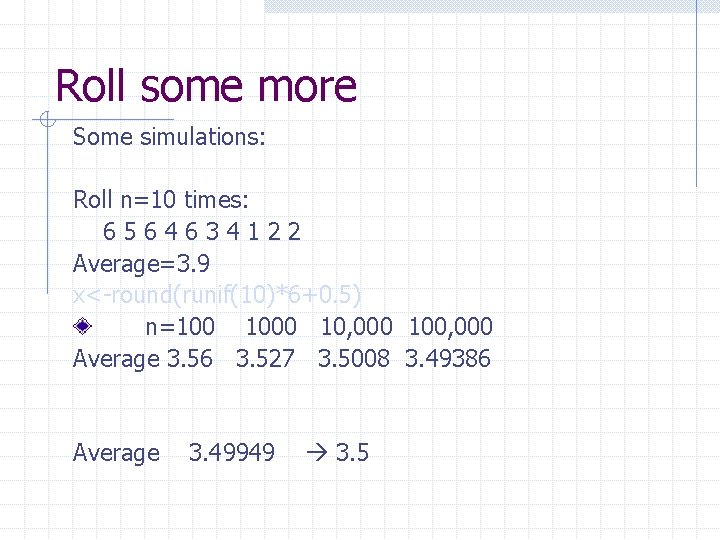Roll some more Some simulations: Roll n=10 times: 6564634122 Average=3. 9 x<-round(runif(10)*6+0. 5) n=100 10, 000 100, 000 Average 3. 56 3. 527 3. 5008 3. 49386 Average 3. 49949 3. 5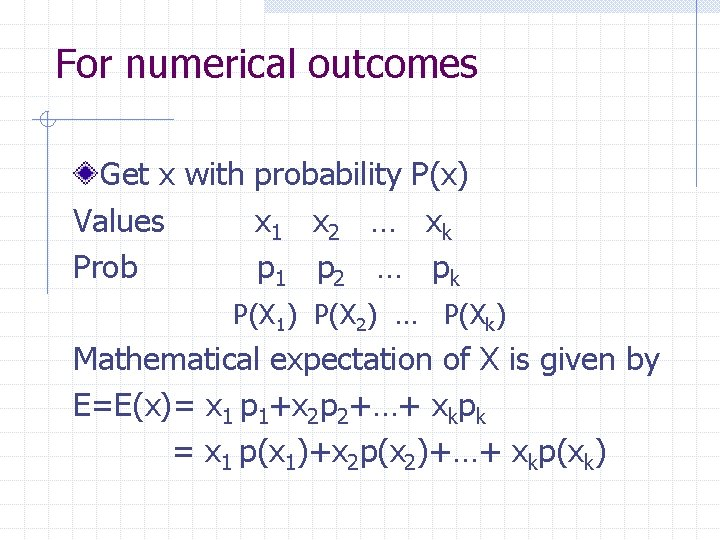For numerical outcomes Get x with probability P(x) Values x 1 x 2 … xk Prob p 1 p 2 … pk P(X 1) P(X 2) … P(Xk) Mathematical expectation of X is given by E=E(x)= x 1 p 1+x 2 p 2+…+ xkpk = x 1 p(x 1)+x 2 p(x 2)+…+ xkp(xk)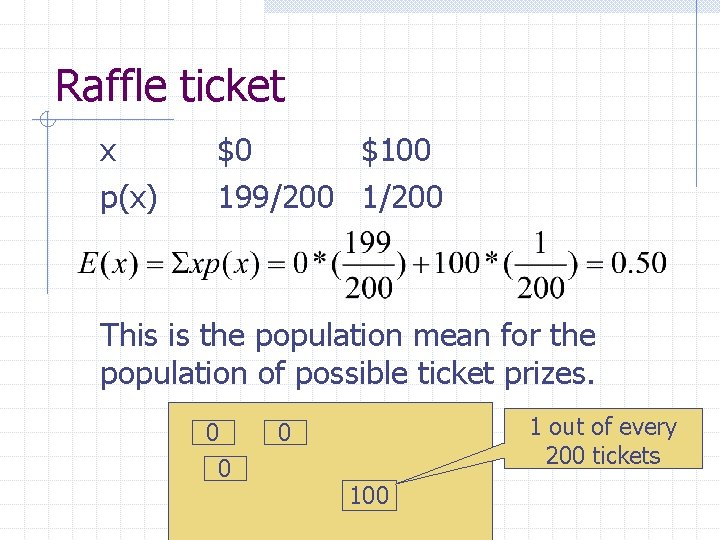Raffle ticket x p(x) \$0 \$100 199/200 1/200 This is the population mean for the population of possible ticket prizes. 0 0 1 out of every 200 tickets 0 100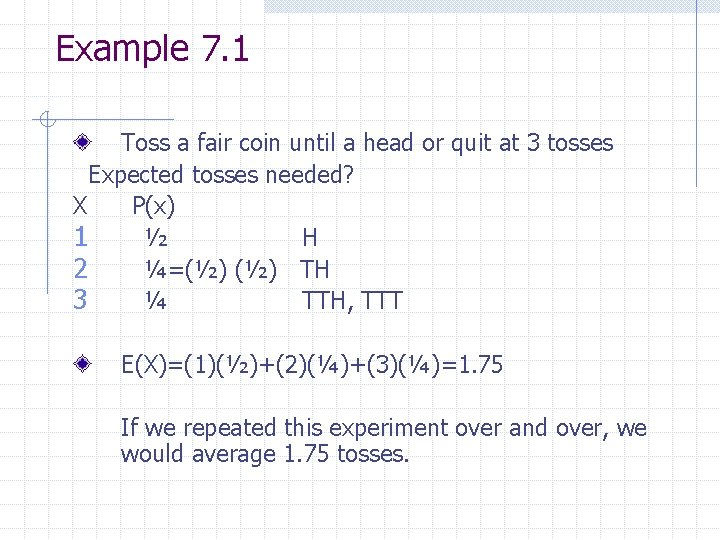Example 7. 1 Toss a fair coin until a head or quit at 3 tosses Expected tosses needed? X P(x) 1 ½ H 2 ¼=(½) TH 3 ¼ TTH, TTT E(X)=(1)(½)+(2)(¼)+(3)(¼)=1. 75 If we repeated this experiment over and over, we would average 1. 75 tosses.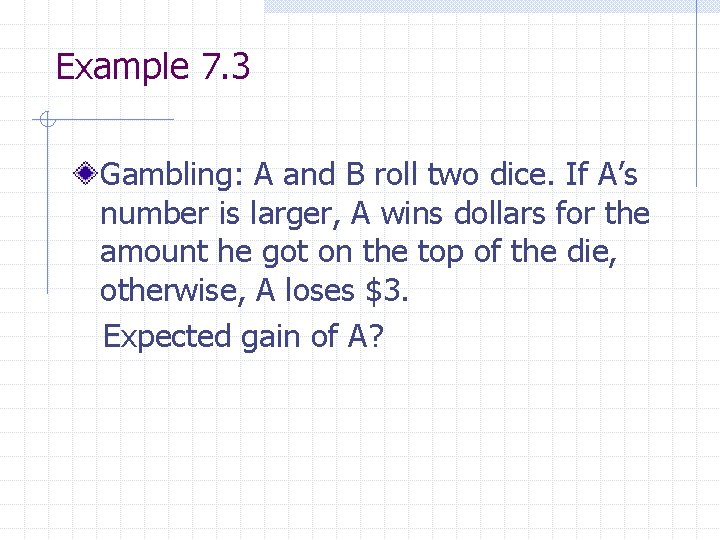Example 7. 3 Gambling: A and B roll two dice. If A’s number is larger, A wins dollars for the amount he got on the top of the die, otherwise, A loses \$3. Expected gain of A?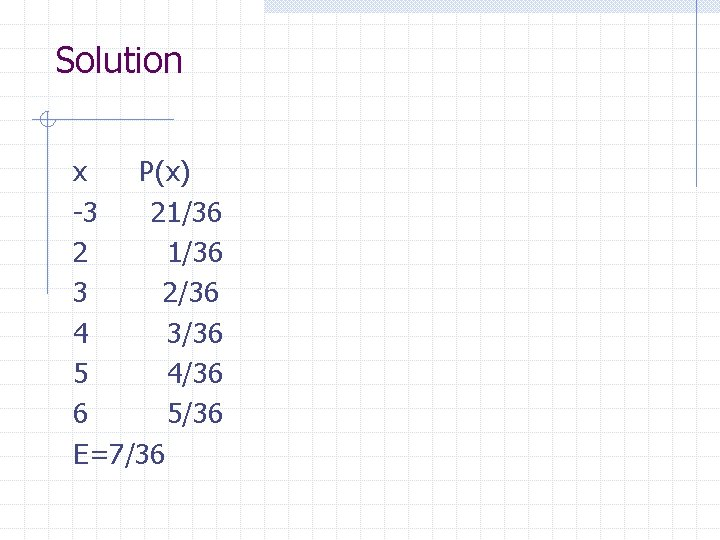Solution x P(x) -3 21/36 2 1/36 3 2/36 4 3/36 5 4/36 6 5/36 E=7/36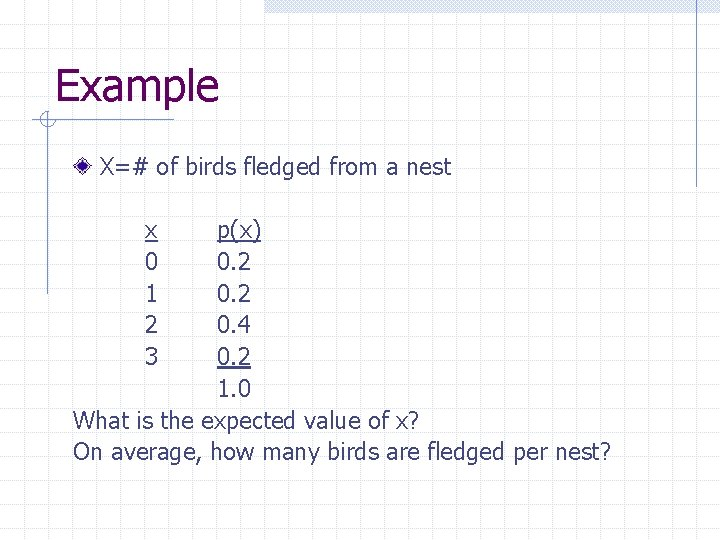Example X=# of birds fledged from a nest x 0 1 2 3 p(x) 0. 2 0. 4 0. 2 1. 0 What is the expected value of x? On average, how many birds are fledged per nest?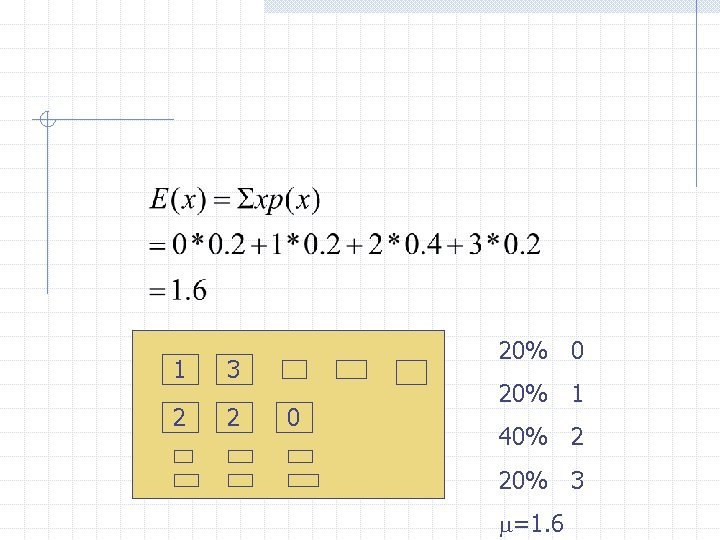1 3 2 2 20% 0 0 20% 1 40% 2 20% 3 m=1. 6